# Example: MHKiT-MATLAB River Module

The following example will familiarize the user with the MHKiT River Module by stepping through the calculation of annual energy produced for one turbine in the Tanana river near Nenana, Alaska. The data file used in this example is retrieved from the USGS website, additional data is stored in the \MHKiT\examples\data directory.

## Importing Data from USGS

We will start by requesting daily discharge data from USGS for the Tanana River near Nenana. The function request_usgs_data in the MHKit river IO module returns a Matlab structure. To use the request_usgs_data function we will need a station number, parameter number, time frame and type of data.
The station ID can be found on the USGS website though this is more difficult than searching the web for "USGS Tanana near Nenana" which should return a link to https://waterdata.usgs.gov/nwis/inventory/?site_no=15515500. This page contains an overview of the available data of most interest in the daily discharge data.
The IEC standard recommends 10 years of daily discharge data for calculations of annual energy produced (AEP). The timeframe shows that at least 10 years of data is available. Clicking on the "Daily Data" will bring up a page that would allow the user to request data from the site. Each of the "Available Parameters" has a 5 digit code in front of it which will depend on the data type and reported units. The user at this point could use the website to download the data, however, here the MHKit request function will be used.
% Use the request method to obtain 10 years of daily discharge data
data=request_usgs_data("15515500",'00060','2009-08-01','2019-08-01',"data_type",'Daily');
Data request URL: https://waterservices.usgs.gov/nwis/dv/?format=json&sites=15515500&startDT=2009-08-01&endDT=2019-08-01&statCd=00003&parameterCd=00060&siteStatus=all
disp(data) % data is a structure with Discharge data
Discharge: [3653×1 double] units: [1×1 struct] time: [1×3653 double]
A Matlab structure was returned with 10 years of daily discharge data. MHKit expects all units to be in SI, so the discharge untis should be converted to m^3/s. We can then plot the discharge time series.
% converting units to m^3/s
data.Discharge = data.Discharge./ (3.28084^3);
data.units = "meters cubed per second"
data = struct with fields:
Discharge: [3653×1 double] units: "meters cubed per second" time: [1×3653 double]
% plot the daily discharge
plot_discharge_timeseries(data);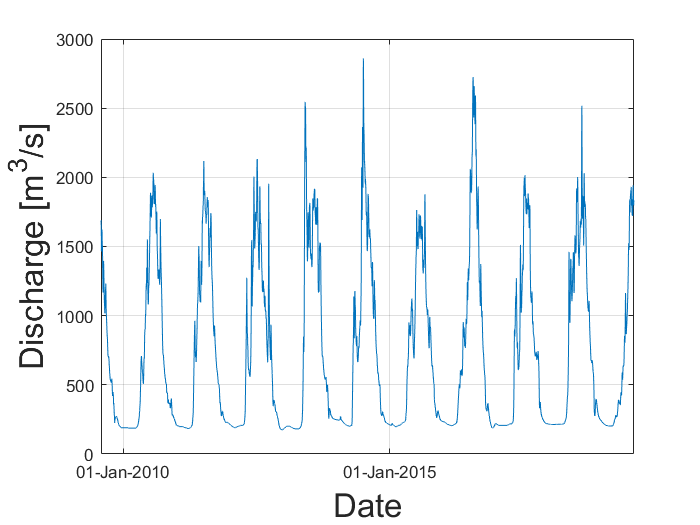## Flow Duration Curve

The flow duration curve (FDC) quantifies the percentage of time that the discharge in a river exceeds a particular magnitude typically compiled on a monthly or annual basis. The FDC is a plot of discharge versus exceedance probability.
The exceedance probability for the highest discharge is close to 0%, rarely being exceeded. Conversely, the lowest flowrates are found closer to 100% as they are most often exceeded.
% Calculating the exceedance probability structure (F) from the Discharge data structure
F = exceedance_probability(data);
% plotting the flow duration curve from Discharge and Exceedance Probability vectors
plot_flow_duration_curve(data.Discharge,F.F);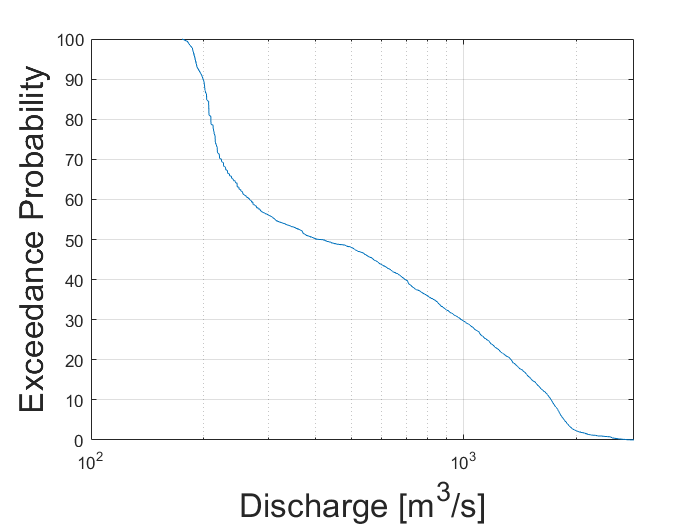## Velocity Duration Curve

At each river energy converter location we must provide a curve that relates the velocity at the turbine location to the river discharge levels. IEC 301 recommends using at least 15 different discharges and that only velocities within the turbine operating conditions need to be included.
Here we only provide 6 different discharge to velocities created using a hydrological model. The data is read into a table from the .csv file.
% Import file with discharge to velocity curve at turbine location
filename = fullfile(pwd,'data/river','tanana_DV_curve.csv');
% Create a polynomial fit of order 3 from the DV_curve.
% The polynomial fit function will return a structure with polynomial fit and R^2 value
p = polynomial_fit(DV_curve.D,DV_curve.V,2); % Input of discharge, velocity, order of fit
% Plot the polynomial curve
plt=plot_discharge_vs_velocity(DV_curve.D,DV_curve.V,"polynomial_coeff",p.coef); % Input of Discharge vector, velocity vector, and polynomial coeficients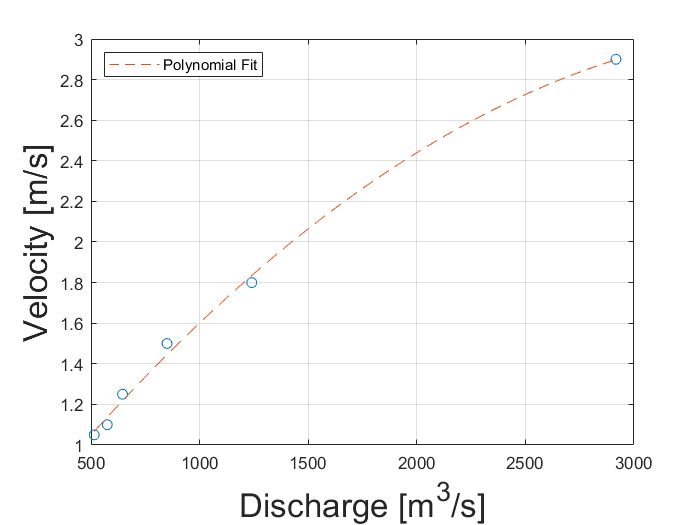The IEC standard recommends a polynomial fit of order 3. By changing the order above and replotting the polynomial fit to the curve data it can be seen this is not a good fit for the provided data. Therefore the order was set to 2.
Next, we can calculate velocity for each discharge in the 10 years years of data using the polynomial and plot the VDC.
% Use polynomial fit from DV curve to calculate velocity('V') from
% discharge at turbine location
V = discharge_to_velocity(data, p.coef); % Input of discharge structure and polynomial coeficients
% Plot the velocity duration curve (VDC)
p2 = plot_velocity_duration_curve(V.V, F.F); % Input velocity vector and exceedance probability vector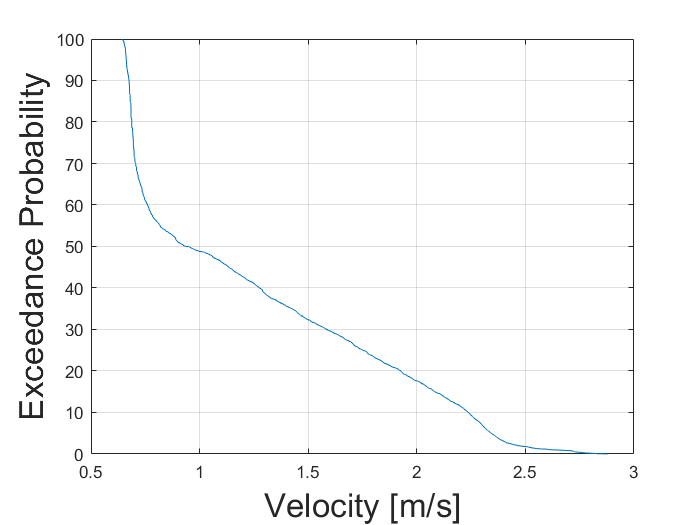## Power Duration Curve

The power duration curve is created in a nearly identical manner to the VDC. Here a velocity to power curve will be used to create a polynomial fit. The polynomial fit will be used to calculate the power produced between the turbine cut-in and cut-out velocities. The velocity to power cure data is loaded into a table from a .csv file.
% We are importing velocity to power data
filename = fullfile(pwd,'data/river','tanana_VP_curve.csv');
% Calculate the polynomial fit for the VP curve
p_VP = polynomial_fit(VP_curve.V, VP_curve.P,2); % Input is vecocity vector, Power vector, and polynomial order
% Plot the VP polynomial curve
p3 = plot_velocity_vs_power(VP_curve.V, VP_curve.P,"polynomial_coeff", p_VP.coef,"title","Velocity vs. Power"); % Input is Velocity vector, Power vector, poly-coef, and Title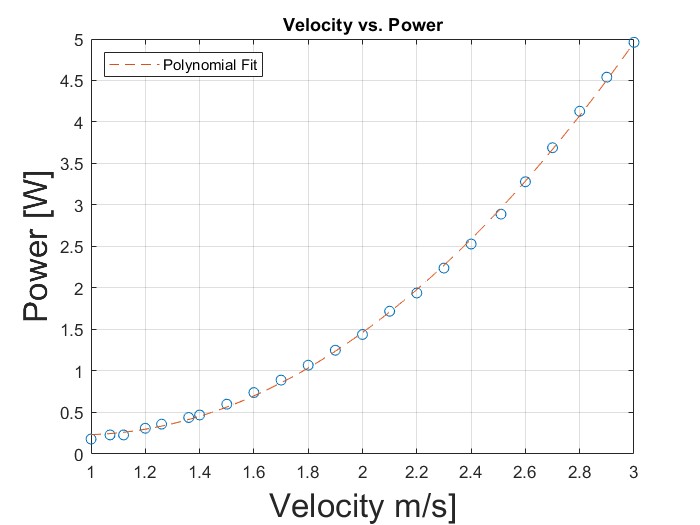% Calculate power from velocity at the turbine location
P = velocity_to_power(V,p_VP.coef,min(VP_curve.V), max(VP_curve.V)); % Input is velocity structure, poly-coef, min and max of VP curve
% Plot the power duration curve
p4 = plot_power_duration_curve(P.P, F.F); % Input of power vector and exceedance probability vector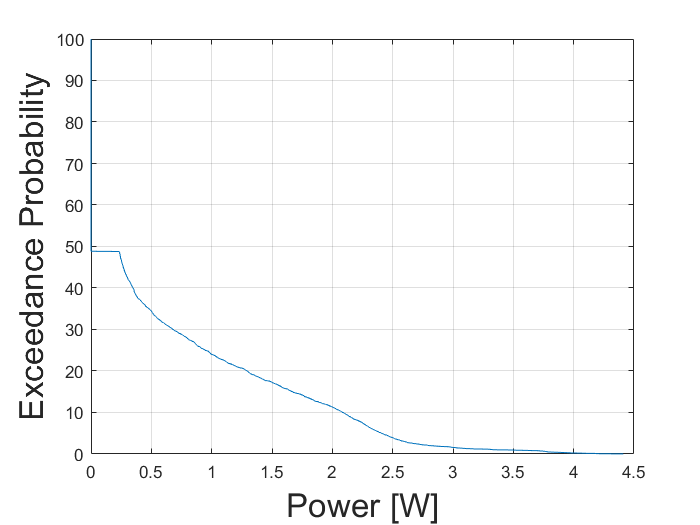## Annual Energy Produced

Finally, annual energy produced is computed, as shown below.
s = 365. * 24 * 3600; % Seconds in a year
% Calculate the Annual Energy produced
AEP = energy_produced(P, s); % Input is exceedance probability structure, Power structure, and number of sec in time period of interest
AEPMW = AEP/1000000; % MW unit conversion
disp('Annual Energy Produced = '+ string(AEPMW) + ' MW')
Annual Energy Produced = 19.0685 MW